### Taylor Series: Applications

#### MacLaurin Series

The following are the MacLaurin series for some basic infinitely differentiable functions: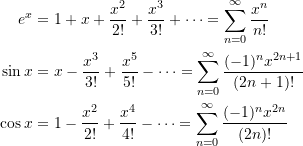#### Numerical Differentiation

Taylor’s Theorem provides a means for approximating the derivatives of a function. The first-order Taylor approximation of a function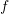is given by: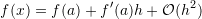where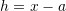is the step size. Then, :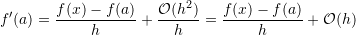##### Forward finite-difference

If we use the Taylor series approximation to estimate the value of the functionat a point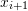knowing the values at point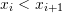then,we have:

(1)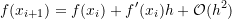where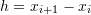. In this case, the forward finite-difference can be used to approximate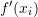as follows: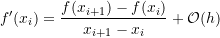##### Backward finite-difference

If we use the Taylor series approximation to estimate the value of the functionat a point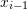knowing the values at point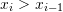then, we have:

(2)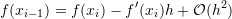where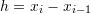. In this case, the backward finite-difference can be used to approximateas follows: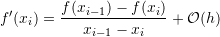##### Centred Finite Difference

The centred finite difference can provide a better estimate for the derivative of a function at a particular point. If the values of a functionare known at the points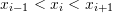and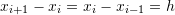, then, we can use the Taylor series to find a good apprxoimation for the derivative as follows: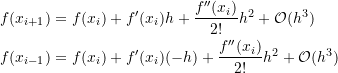Subtracting the above two equations and dividing by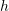gives the following: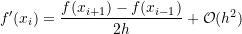#### Building Differential Equations

See momentum balance and beam approximation for examples where the Taylor’s approximation is used to build a differential equation.

#### Approximating Continuous Functions

Taylor’s theorem essentially discusses approximating differentiable functions using polynomials. The approximation can be as close as needed by adding more polynomial terms and/or by ensuring that the step sizeis small enough. It is important to realize that polynomial approximations are valid for continuous functions that are not necessarily differentiable at every point. Let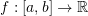, then the Stone-Weierstrass Theorem states that for any small number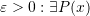a polynomial function such that the biggest difference between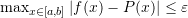. In other words, we can always find a polynomial function that approximates any continuous function on the interval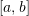within any degree of accuracy sought! This fact is extremely important in all engineering applications. Essentially, it allows modelling any continuous functions using polynomials. In other words, if a problem wishes to find the distribution of a variable (stress, strain, velocity, density, etc…) as a function of position, and if this variable is continuous, then, in the majority of applications we can assume differentiability and a Taylor approximation for the unknown function.